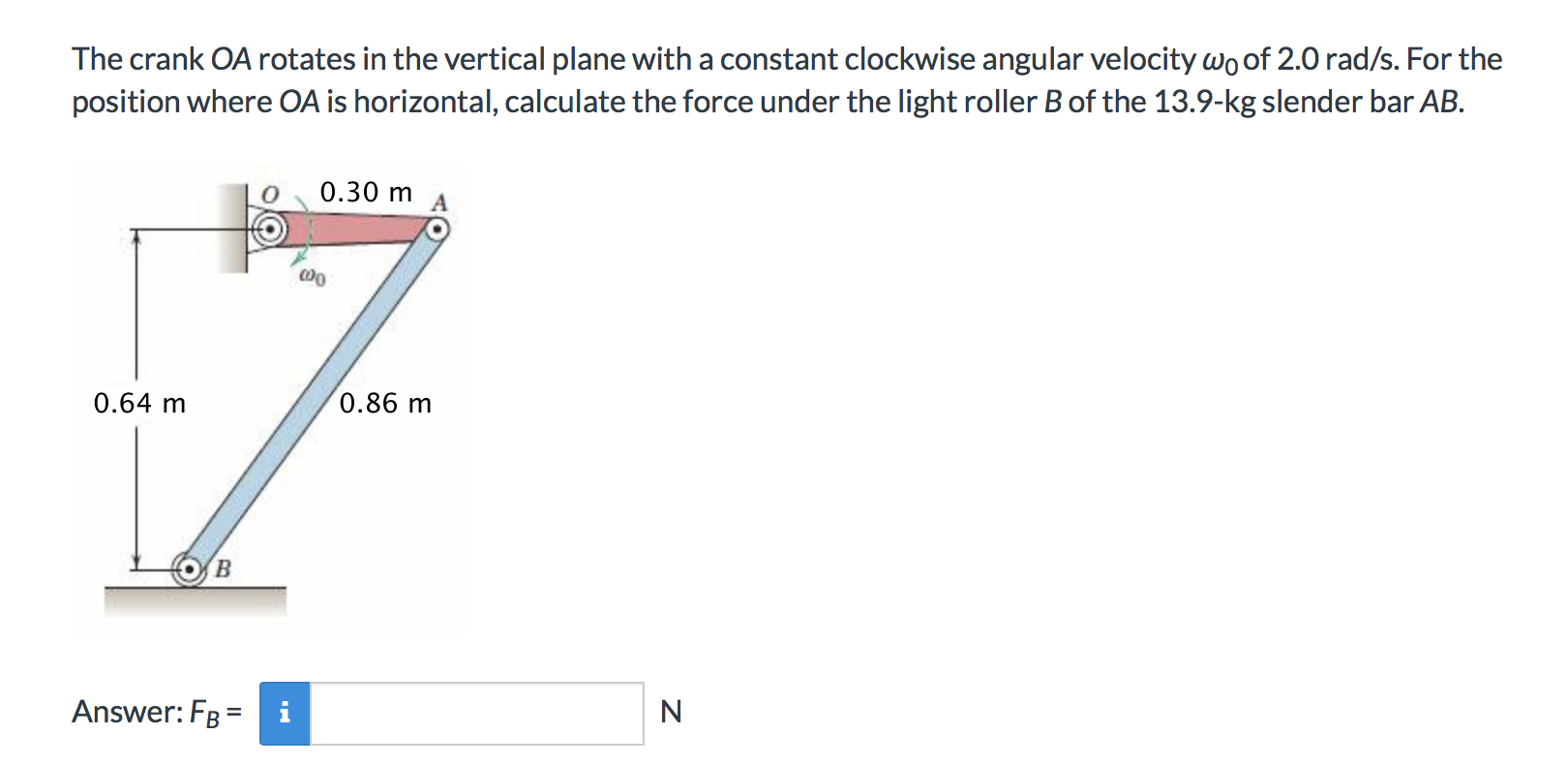# The crank OA rotates in the vertical plane with a constant clockwise angular velocity wo of 2.0 rad/s. For theposition where OA is horizontal, calculate the force under the light roller B of the 13.9-kg slender bar AB.0.30 m0.86 m0.64 mAnswer: FBNi

Question
472 viewshelp_outlineImage TranscriptioncloseThe crank OA rotates in the vertical plane with a constant clockwise angular velocity wo of 2.0 rad/s. For the position where OA is horizontal, calculate the force under the light roller B of the 13.9-kg slender bar AB. 0.30 m 0.86 m 0.64 m Answer: FB N i fullscreen
check_circle

Step 1

Consider the clockwise sense of rotation as positive and write the expression for magnitude of velocity of point A which will be directed downwards. Further, substitute suitable values to evaluate the same as:

Step 2

Now, consider the Instantaneous center of the bar AB as an arbitrary point C as shown in the figure. Write the expression for magnitude of angular velocity of the bar which will be in clockwise. Further, substitute suitable values to evaluate the same as:

Step 3

Since the crank is rotating with constant angular velocity, point A will only experience radial component of acceleration directed towards point O. Write the ex...

### Want to see the full answer?

See Solution

#### Want to see this answer and more?

Solutions are written by subject experts who are available 24/7. Questions are typically answered within 1 hour.*

See Solution
*Response times may vary by subject and question.
Tagged in